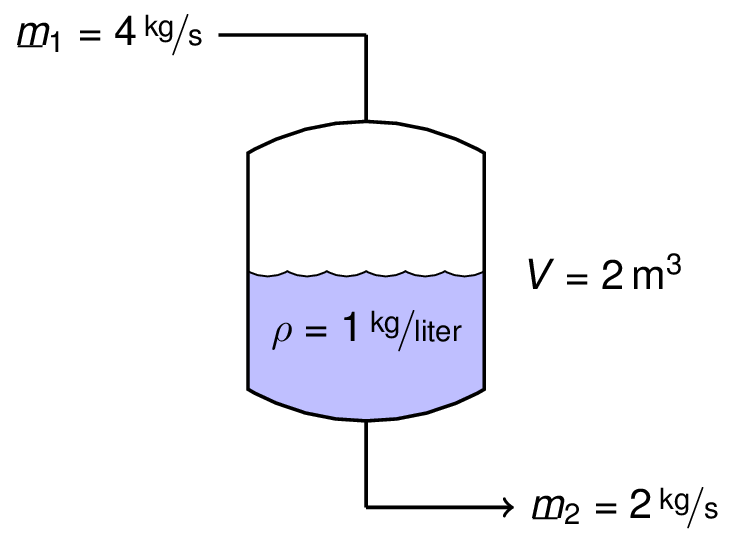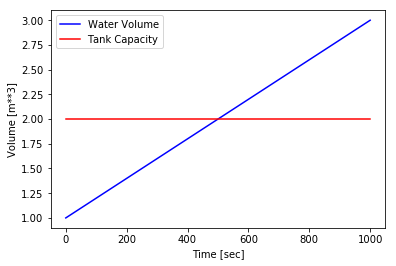This notebook contains course material from CBE20255 by Jeffrey Kantor (jeff at nd.edu); the content is available on Github. The text is released under the CC-BY-NC-ND-4.0 license, and code is released under the MIT license.

# General Mass Balance on a Single Tank¶

## Summary¶

This Jupyter notebook demonstrates the application of a mass balance to a simple water tank. This example is adapted with permission from learnCheme.com, a project at the University of Colorado funded by the National Science Foundation and the Shell Corporation.

## Problem Statement¶

### Draw a Diagram¶### Mass Balance¶

Using our general principles for a mass balance

$\frac{d(\rho V)}{dt} = \dot{m}_1 - \dot{m}_2$

which can be simplified to

$\frac{dV}{dt} = \frac{1}{\rho}\left(\dot{m}_1 - \dot{m}_2\right)$

where the initial value is $V(0) = 1\,\mbox{m}^3$. This is a differential equation.

### Numerical Solution using odeint¶

There are a number of numerical methods available for solving differential equations. Here we use odeint which is part of the scipy package. odeint requires a function that returns the rate of accumulation in the tank as a function of the current volume and time.

In :
import numpy as np
import matplotlib.pyplot as plt
%matplotlib inline

from scipy.integrate import odeint

In :
# Flowrates in kg/sec
m1 = 4.0
m2 = 2.0

# Density in kg/m**3
rho = 1000.0

# Function to compute accumulation rate
def dV(V,t): return (m1 - m2)/rho;


Next we import odeint from the scipy.integrate package, set up a grid of times at which we wish to find solution values, then call odeint to compute values for the solution starting with an initial condition of 1.0.

In :
t = np.linspace(0,1000)
V = odeint(dV,1.0,t)


We finish by plotting the results of the integration and comparing to the capacity of the tank.

In :
plt.plot(t,V,'b',t,2*np.ones(len(t)),'r')
plt.xlabel('Time [sec]')
plt.ylabel('Volume [m**3]')
plt.legend(['Water Volume','Tank Capacity'],loc='upper left');This same approach can be used solve systems of differential equations. For an light-hearted (but very useful) example, check out this solution for the Zombie Apocalypse.

### Solving for the Time Required to Fill the Tank¶

Now that we know how to solve the differential equation, next we create a function to compute the air volume of the tank at any given time.

In :
Vtank = 2.0
Vinitial = 1.0

def Vwater(t):
return odeint(dV,Vinitial,[0,t])[-1]

def Vair(t):
return Vtank - Vwater(t)

print("Air volume in the tank at t = 100 is {:4.2f} m**3.".format(Vair(100)))

Air volume in the tank at t = 100 is 0.80 m**3.


The next step is find the time at which Vair(t) returns a value of 0. This is root finding which the function brentq will do for us.

In :
from scipy.optimize import brentq

t_full = brentq(Vair,0,1000)

print("The tank will be full at t = {:6.2f} seconds.".format(t_full))

The tank will be full at t = 500.00 seconds.


## Exercise¶

Suppose the tank was being used to protect against surges in water flow, and the inlet flowrate was a function of time where

$\dot{m}_1 = 4 e^{-t/500}$

• Will the tank overflow?
• Assuming it doesn't overflow, how long would it take for the tank to return to its initial condition of being half full? To empty completely?
• What will be the peak volume of water in the tank, and when will that occur?
In [ ]: